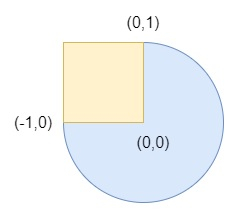# Circle and Rectangle Overlapping in C++

Suppose we have a circle represented as (radius, xc, yc), here (xc, yc) is the center coordinate of the circle. We also have an axis-aligned rectangle represented as (x1, y1, x2, y2), where (x1, y1) are the coordinates of the bottom-left corner, and (x2, y2) are the coordinates of the top-right corner of the rectangle. We have to check whether the circle and rectangle are overlapped or not.

So, if the input is likethen the output will be true.

To solve this, we will follow these steps −

• Define a function eval(), this will take a, b, c,

• return maximum of b and minimum of a and c

• From the main method, do the following −

• cdx := eval(cx, left, right), cdy := eval(cy, bottom, top)

• rwid := right - left, rh := top - bottom

• dx := cx - cdx, dy := cy - cdy

• disSq := (dx * dx) + (dy * dy)

• sqrRadius := (r * r)

• return true when disSq <= sqrRadius, otherwise false

## Example

Let us see the following implementation to get better understanding −

Live Demo

#include <bits/stdc++.h>
using namespace std;
class Solution {
public:
int eval(int a, int b, int c){
return max(b, min(a, c));
}
bool checkOverlap(int r, int cx, int cy, int left, int bottom, int right, int top){
double cdx = eval(cx, left, right);
double cdy = eval(cy, bottom, top);
double rwid = right - left;
double rh = top - bottom;
double dx = cx - cdx;
double dy = cy - cdy;
double disSq = (dx * dx) + (dy * dy);
double sqrRadius = (r * r);
}
};
main(){
Solution ob;
cout << (ob.checkOverlap(1, 0, 0, 1, -1, 3, 1));
}

## Input

1, 0, 0, 1, -1, 3, 1

## Output

1